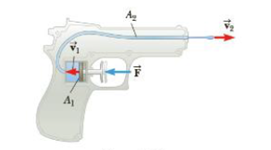Chapter 9, Problem 41P

Chapter
Section
Textbook Problem

In a water pistol, a piston drives water through a larger tube of radius 1.00 cm into a smaller tube of radius 1.00 mm as in Figure P9.41. (a) If the pistol is fired horizontally at a height of 1.50 m, use ballistics to determine the time it takes water to travel from the nozzle to the ground. (Neglect air resistance and assume atmospheric pressure is 1.00 atm.) (b) If the range of the stream is to be 8.00 m, with what speed must, the stream lease the nozzle? (c) Given the areas of the nozzle and cylinder, use the equation of continuity to calculate the speed at which the plunger must be moved, (d) What is the pressure at the nozzle? (e) Use Bernoulli’s equation to find the pressure needed in the larger cylinder. Can gravity terms lie neglected? (f) Calculate the force that must be exerted on the trigger to achieve the desired range. (The force dial must be exerted is due to pressure over and above atmospheric pressure.)Figure P9.41

(a)

To determine
The time taken by the water to travel from the nozzle to the ground.

Explanation

Formula to calculate the time taken by the water to reach the ground is,

t=2(Δy)ay

• t is taken time,
• (Δy) is the distance
• ay is the acceleration along upward direction.

Substitute (1.5m) for (Δy) , (9

(b)

To determine
The speed that must have the stream while leaving the nozzle.

(c)

To determine
The speed at which the plunger must be moved by using continuity equation.

(d)

To determine
The pressure of the nozzle.

(e)

To determine
The pressure needed in the larger cylinder by using Bernoulli’s equation.

(f)

To determine
The force that must be exerted on the trigger to achieve the desired range.

Still sussing out bartleby?

Check out a sample textbook solution.

See a sample solution

The Solution to Your Study Problems

Bartleby provides explanations to thousands of textbook problems written by our experts, many with advanced degrees!

Get Started

Find more solutions based on key concepts